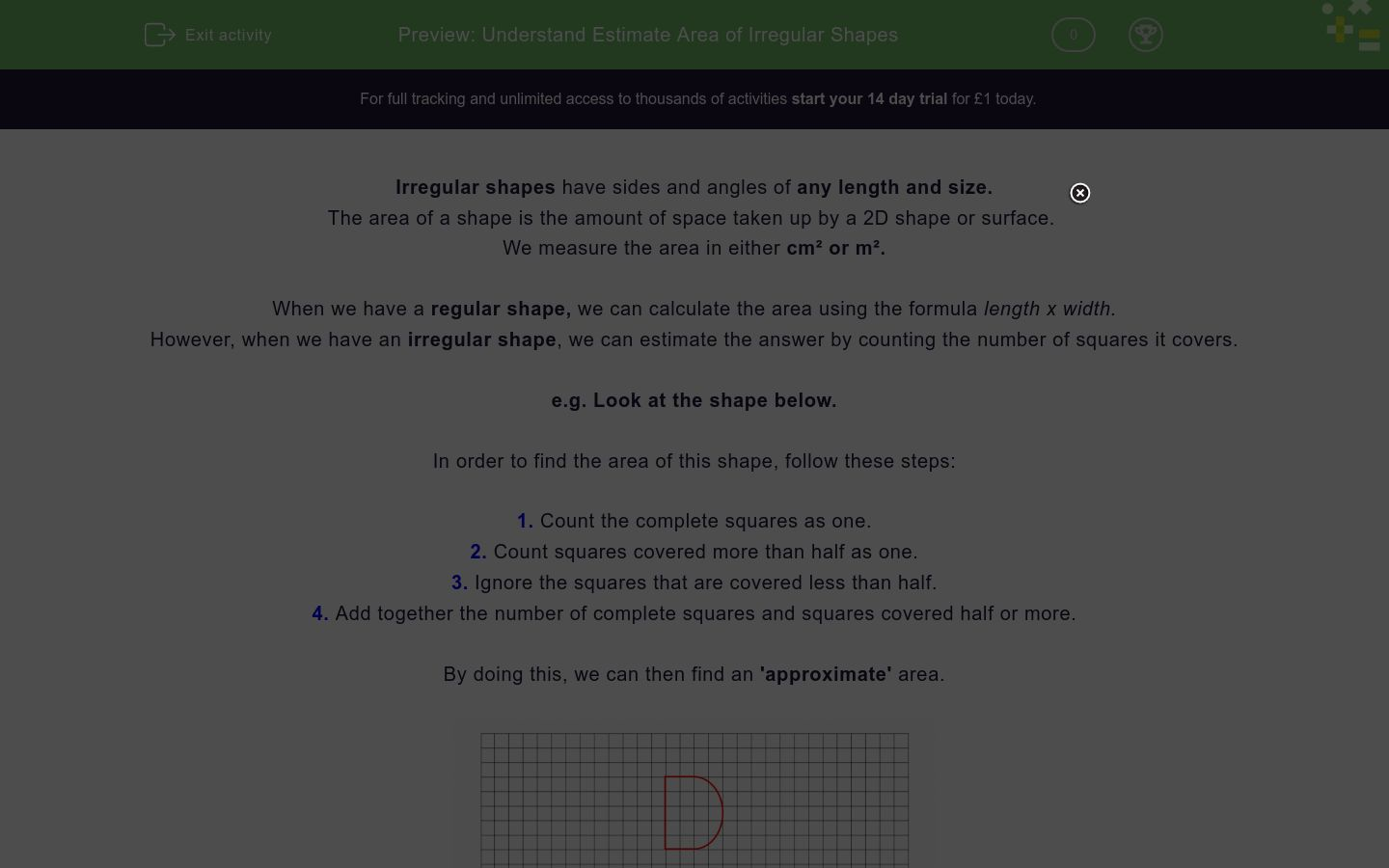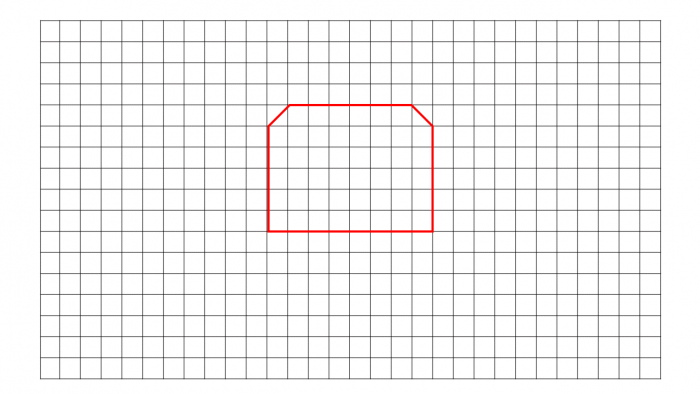# Understand Estimate Area of Irregular Shapes

In this worksheet, students will be asked to calculate the estimated area of irregular shapes. Students will use a cm square grid in order to find the area.Key stage:  KS 2

Curriculum topic:   Measurement

Curriculum subtopic:   Calculate/Estimate Areas

Difficulty level:### QUESTION 1 of 10

Irregular shapes have sides and angles of any length and size.

The area of a shape is the amount of space taken up by a 2D shape or surface.

We measure the area in either cm² or m².

When we have a regular shape, we can calculate the area using the formula length x width.

However, when we have an irregular shape, we can estimate the answer by counting the number of squares it covers.

e.g. Look at the shape below.

In order to find the area of this shape, follow these steps:

1. Count the complete squares as one.

2. Count squares covered more than half as one.

3. Ignore the squares that are covered less than half.

4. Add together the number of complete squares and squares covered half or more.

By doing this, we can then find an 'approximate' area.Complete squares = 13

Squares covered more than half = 5

Approx Total Area = 18 cm²

Want to understand this further and learn how this links to other topics in maths?

Why not watch this video?

Look at the irregular shape below.

Count the number of whole squares, count the number of squares that are half or more covered and add together.

Choose the correct number for the total area in cm².21.5 cm²

24 cm²

23 cm²

22 cm²

Look at the irregular shape below.

Count the number of whole squares, count the number of squares that are half or more covered and add together.

Choose the correct number for the total area in cm².28 cm²

30 cm²

28.5 cm²

29 cm²

Look at the irregular shape below.

Count the number of whole squares, count the number of squares that are half or more covered and add together.

Choose the correct number for the total area in cm².22 cm²

23.5 cm²

24 cm²

23 cm²

Look at the irregular shape below.

Count the number of whole squares, count the number of squares that are half or more covered and add together.

Write the correct number for the total area in cm².22 cm²

23.5 cm²

24 cm²

23 cm²

Look at the irregular shape below.

Count the number of whole squares, count the number of squares that are half or more covered and add together.

Write the correct number for the total area in cm².22 cm²

23.5 cm²

24 cm²

23 cm²

Look at the irregular shape below.

Count the number of whole squares, count the number of squares that are half or more covered and add together.

Write the correct number for the total area in cm².22 cm²

23.5 cm²

24 cm²

23 cm²

Look at the irregular shapes below.

Below, choose which shape has the biggest approximate area.

Shape AShape BShape A

Shape B

Look at the irregular shapes below.

Below, choose which shape has the biggest approximate area.

Shape AShape BShape A

Shape B

When finding the area of an irregular shape, how do you count the squares?

Count all squares as one

Count complete squares as one, count squares covered half or more as one and ignore other squares

Count complete squares as one and ignore the rest

Count complete squares as one and count all other squares as half

James looks at the irregular shape below and states that its area is approximately 24 cm².Correct

Incorrect

• Question 1

Look at the irregular shape below.

Count the number of whole squares, count the number of squares that are half or more covered and add together.

Choose the correct number for the total area in cm².24 cm²
EDDIE SAYS
You have to count carefully when finding the area of tricky, irregular shapes. This shape has 19 whole squares and 5 squares that are half or more covered. This gives us the approximate area of 24 cm². Pretty simple, right?
• Question 2

Look at the irregular shape below.

Count the number of whole squares, count the number of squares that are half or more covered and add together.

Choose the correct number for the total area in cm².29 cm²
EDDIE SAYS
Again, you have to count carefully when finding the area of tricky, irregular shapes. This shape has 27 whole squares and 2 squares that are half or more covered. This gives us the approximate area of 29 cm².
• Question 3

Look at the irregular shape below.

Count the number of whole squares, count the number of squares that are half or more covered and add together.

Choose the correct number for the total area in cm².23 cm²
EDDIE SAYS
How did you get on this time counting the squares? Make sure you always check your answer. This shape has 18 whole squares and 5 squares that are half or more covered. This gives us the approximate area of 23 cm².
• Question 4

Look at the irregular shape below.

Count the number of whole squares, count the number of squares that are half or more covered and add together.

Write the correct number for the total area in cm².EDDIE SAYS
How did you find it this time without any options to choose from? This shape has 24 whole squares and 3 squares that are half or more covered. This give us the approximate area of 27 cm².
• Question 5

Look at the irregular shape below.

Count the number of whole squares, count the number of squares that are half or more covered and add together.

Write the correct number for the total area in cm².EDDIE SAYS
This shape has 46 whole squares and 2 squares that are half or more covered. This gives us the approximate area of 48 cm².
• Question 6

Look at the irregular shape below.

Count the number of whole squares, count the number of squares that are half or more covered and add together.

Write the correct number for the total area in cm².EDDIE SAYS
This shape has 14 whole squares and 14 squares that are half or more covered. This gives us the approximate area of 28 cm².
• Question 7

Look at the irregular shapes below.

Below, choose which shape has the biggest approximate area.

Shape AShape BShape A
EDDIE SAYS
Shape A has an approximate area of 37 cm². Shape B has an approximate area of 27 cm². Therefore, Shape A has the largest area.
• Question 8

Look at the irregular shapes below.

Below, choose which shape has the biggest approximate area.

Shape AShape BShape B
EDDIE SAYS
Shape A has an approximate area of 18 cm². Shape B has an approximate area of 23 cm². Therefore, Shape B has the largest area. Great progress, you've only got two more questions to complete!
• Question 9

When finding the area of an irregular shape, how do you count the squares?

Count complete squares as one, count squares covered half or more as one and ignore other squares
EDDIE SAYS
It is important to remember that when calculating the area of an irregular shape, we follow the following rules 1. Count all complete squares as one. 2. Count squares covered half or more as one. 3. Ignore squares covered less than half. 4. Add the total from step 1 and 2 to find the approximate area. You should be a pro at this by now, maths-whiz!
• Question 10

James looks at the irregular shape below and states that its area is approximately 24 cm².Incorrect
EDDIE SAYS
Unfortunately, James is incorrect. This irregular shape has 22 whole squares covered and 4 squares that are covered half or more, giving it an approximate area of 26 cm². Incredible work. Why not attempt the next activity and then take a well deserved break?
---- OR ----

Sign up for a £1 trial so you can track and measure your child's progress on this activity.

### What is EdPlace?

We're your National Curriculum aligned online education content provider helping each child succeed in English, maths and science from year 1 to GCSE. With an EdPlace account you’ll be able to track and measure progress, helping each child achieve their best. We build confidence and attainment by personalising each child’s learning at a level that suits them.

Get started